# Online Test For Class 11 Physics Laws of Motion

Practice class 11 physics chapter 5 Laws of Motion objective type questions through this online test. This is a very important chapter for preparation of competitive exams point of view.

## Laws of Motion Online MCQ Test

General Instructions

1. Your test contains multiple-choice questions with only one answer type questions. There are a total of 25 questions
2. This is a 45 min test. Please make sure you complete it in stipulated time
3. You can finish this test any time using the 'Submit' button.

1. Two particles of masses $m_1$ and $m_2$ in projectile motion have velocities $\vec{v_1}<\vec{v_2}$ respectively at time $t=0$. Thet collide at time $t_0$ . Their velocities become $\vec{v'_1}$ and $\vec{v'_2}$ at time $2t_0$, while still moving in air. The value of $\left|\left(m_1\vec{v'_1}+m_2\vec{v'_2}\right)-\left(m_1\vec{v_1}+m_2\vec{v_2}\right)\right|$ is
2. A string of negligible mass going over clamped pully of mass supports block of mass as shown in the figure.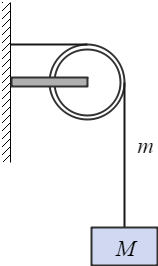The force on the pully by clamp is given by
3. When a bicycle is in motion, the force of friction exerted by ground on the two wheels is such that, it acts
4. A rocket with a lift-off mass $3.5\times{10}^4Kg$ is blasted upwards with an initial acceleration of $10m/s^2$. Then the initial thrust blast is
5. 5 The block of mass $M$ is pulled along a horizontal frictionless surface by a rope of mass $m$. If a force $P$ is applied at the free end of the rope, the force exerted by the rope on the block is
6. Three forces start action simultaneously on a particle moving with velocity $\vec{v}$. These forces are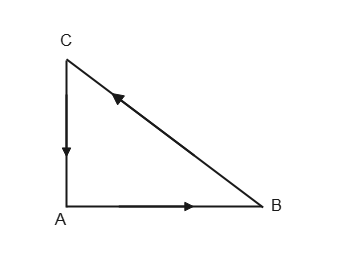Represented in magnitude and direction by the three sides of a triangle $ABC$ as shown in the figure. The particle will now move with velocity
7. A lift is moving down with acceleration a. A man in the lift drops a ball inside the lift. The acceleration of the ball is observed by the man in the lift and a man standing stationary on the ground are respectively.
8. A light spring balance hangs from the hook of the other light spring balance and a block of mass $M\, Kg$ hangs from the former one. Then the true statement about the scale reading is
9. A block rests on a rough inclined plane making an angle of ${30}^0$ with the horizontal. The coefficient of static friction between the block and the plane is $0.8$. If the frictional force on the block is $10\, N$, the mass of the block (in Kg) $\left(g=10m/s^2\right)$ is
10. A horizontal force of  is necessary to just hold a block stationary against a wall. The coefficient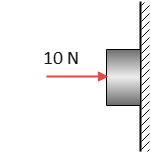Of friction between the block is 0.2. The weight of the block is
11. A smooth block is released at rest on a ${45}^0$ incline and then slides a distance $d$. The time taken to slide is $n$ times as much to slide on a rough incline than on a smooth incline. The coefficient of friction is
12. A particle is acted upon by a force of constant magnitude which is always perpendicular to the velocity of the particle. The motion of the particle takes place in a plane. It follows that
13. An annular ring with inner and outer radii $R_1$ and $R_2$ is rolling without slipping with a uniform angular speed. The ratio of the forces $F_1/F_2$ experienced by two identical particles situated on the inner and outer parts of the ring is
14. Two frictionless inclined planes making angles ${30}^0$ and ${60}^0$ with the vertical are shown in the figure. Two blocks A and B are placed on two planes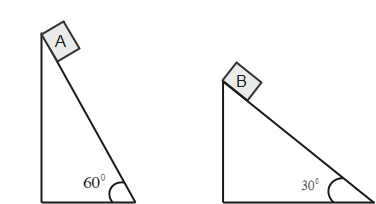What is the relative vertical acceleration of A with respect to B?
15. Question 15 Which of the four arrangements in the figure correctly shows the vector addition of two forces $F_1$ and $F_2$ to yield the third force $F_3$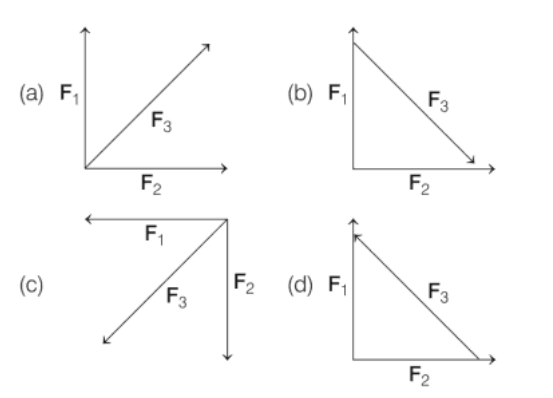16. A body of mass 5 Kg starts from the origin with an initial velocity $\vec{u}=\left(30\hat{i}+40\hat{j}\right) m/s$. If a constant force $\left(-6\hat{i}-5\hat{j}\right)N$ acts on the body, the time in which y component of velocity becomes zero is
17. Three blocks of mass 2 Kg, 3Kg, 5 Kg are connected to each other with a light string and are then placed on a frictionless surface as shown in the figure. The system is pulled by a force $F=10\, N$, then tension $T_1$ is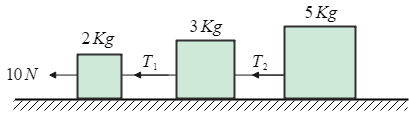18. A particle is moving in a circle with uniform speed $v$. In moving from a point to another diametrically opposite point,
19. A person is sitting in a lift accelerating upwards. The measured weight of a person will be,
20. Which of the following statements is true?
21. In following question, a statement of assertion is followed by a statement of reason. Mark the correct choice.
Assertion: A rocket moves forward by pushing surrounding air backwards.
Reason: It derives the necessary thrust to move forward, according to Newton’s third law of motion.
22. In following question, a statement of assertion is followed by a statement of reason. Mark the correct choice.
Assertion: Frictional forces are conservative forces.
Reason: Potential energy can be associated with frictional forces
23. In following question, a statement of assertion is followed by a statement of reason. Mark the correct choice.
Assertion: The driver in a vehicle moving with a constant speed on a straight road is a non-inertial frame of reference.
Reason: A reference frame, in which Newton’s laws of motion are applicable are, is non inertial.
24. In following question, a statement of assertion is followed by a statement of reason. Mark the correct choice.
Assertion: A man in a closed cabin, which is falling freely, does not experience gravity.
Reason: Inertial and gravitational masses have equivalence.
25. In following question, a statement of assertion is followed by a statement of reason. Mark the correct choice.
Assertion: It is difficult to move a cycle along the road with its break on.
Reason: Sliding friction is greater than rolling friction.

Subscribe
Notify ofThis site uses Akismet to reduce spam. Learn how your comment data is processed.

1 Comment
Inline Feedbacks